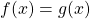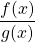# 2. Polynomial and Rational Functions

 1 Prerequisites 2 Introduction 3 The factored form and the factor theorem 4 Solving5 Graphing, Point Discontinuity 6 Graphing, Linear/Linear 7 Graphing, Other/Linear and the Remainder Theorem

Quiz 1 Practice Properties of Polynomials

Quiz 2 Practice Remainder and Factor Theorems

Quiz 3 Practice Rational Functions

# Unit Review

Grade 12 Precalc Unit 2 Review